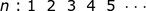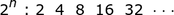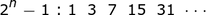# Perfect Numbers & Mersenne Primes

Instructor: Gerald Lemay

Gerald has taught engineering, math and science and has a doctorate in electrical engineering.

Although computers facilitate searches for prime numbers and perfect numbers, fundamental number theory is still very relevant. In this lesson we explore how a particular type of prime number, the Mersenne prime, relates to perfect numbers.

## Playing With Perfect Numbers

Searching for perfect numbers and prime numbers began thousands of years ago and continues to this day. Perfect numbers and prime numbers are uniquely defined by their divisors. Some of the prime numbers discovered are Mersenne primes. These primes are linked to powers of 2. In this lesson we explore Mersenne primes and their fascinating relationship with perfect numbers.

Just for fun, add the divisors of 6 not including the 6. Adding 1 plus 2 plus 3 gives 6. Six is the starting number and 6 is the sum. Six is a perfect number where the sum of the divisors (not including the number itself) equals the number.

What about the number 8?

First, find the divisors of 8. The divisors of 8 are 1, 2, 4 and 8. Is 8 a perfect number? Adding 1 + 2 + 4 gives 7 which does not equal 8. Thus, 8 is not a perfect number.

How about 3? The divisors of 3 are 1 and 3. Not a perfect number either but 3 is a prime number, in that it has only two divisors: the number itself and 1.

Could a prime number ever be a perfect number? What is the divisor sum if the number is a prime number? Right, always a 1. Thus, none of the prime numbers are perfect numbers.

Ready for another idea? There's a special kind of prime number called the Mersenne prime.

## Finding Mersenne Primes

Mersenne primes are prime numbers that are one less than a power of 2; compactly written as 2n - 1. A systematic way to find Mersenne primes:

• calculate 2n for n = 1, 2, 3, ....
• subtract 1
• check for prime numbers

Instead of doing a thorough search right away, let's do n = 2.

Step 1, calculate 2n for n = 2. Thus, 22 = 2(2) = 4.

Step 2, subtract 1 from 4 which is 3.

Step 3, check if 3 is prime. The divisors of 3 are only 3 and 1. Thus, 3 is prime.

Conclusion: 3 is a Mersenne prime.

Do all prime numbers have the 2n - 1 recipe? How about the prime number 5? Well, 5 is prime because the divisors are 5 and 1. But there no n for the 2n - 1 would result in 5. Although 5 is prime number, 5 is not a Mersenne prime.

Ready for another idea? Mersenne primes can be used to find perfect numbers.

## Using Mersenne Primes to Find Perfect Numbers

Having a Mersenne prime, the n is known. With this n we find a perfect number using the formula 2n-1 (2n - 1). This formula contains the 2n - 1 Mersenne primes with a 2n-1 multiplier in front.

Remember the perfect number, 6, and the Mersenne prime, 3? The n was 2 in 2n - 1 to get the 3. Now, use this n = 2 in 2n-1 (2n - 1) to get a perfect number.

22-1 (22 - 1) = 21 (4 - 1) = 2(3) = 6. There's our perfect number, 6.

Let's find the first 3 Mersenne primes using a systematic search:

• Write some increasing values for n starting with n = 1:• Calculate 2n for each of these values for n:For example, for n = 5, 25 is 2(2)(2)(2)(2) = 2(4)(4) = 2(16) = 32.

• Subtract 1 from each result:• From this list, pick out the prime numbers:

3 7 31 … are the prime numbers. The number 15 has more than two divisors, and so is not prime. Note: 1 is not prime because it has only one divisor.

To unlock this lesson you must be a Study.com Member.

### Register to view this lesson

Are you a student or a teacher?

### Unlock Your Education

#### See for yourself why 30 million people use Study.com

##### Become a Study.com member and start learning now.
Back
What teachers are saying about Study.com

### Earning College Credit

Did you know… We have over 160 college courses that prepare you to earn credit by exam that is accepted by over 1,500 colleges and universities. You can test out of the first two years of college and save thousands off your degree. Anyone can earn credit-by-exam regardless of age or education level.